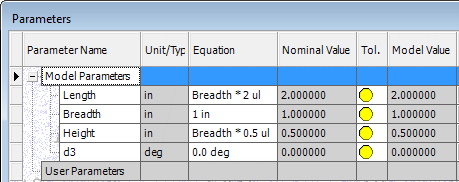# Autodesk Inventor 2011 (Part 2) – iLogic

<< Part 1

In March 2009 Autodesk released a special add-in for its Inventor subscription customers called iLogic that allowed a greater level of intelligence to be built into Inventor models, much more than the intelligence that parametric modeling already offers. In Inventor 2011, the iLogic add-in has become part of the core product. In this part of the series I will try and give a very brief introduction of iLogic. In the next part I will discuss the new features that have been added to it in Inventor 2011.

iLogic was the result of an acquisition that Autodesk made of a company called Logimetrix back in 2008. Basically the iLogic add-in bridges the gap between parametric modeling and knowledge based engineering. Complex concepts are best explained by means of simple examples. And so I will proceed to do exactly that. Say we extrude a rectangle to get a box in Inventor. In doing so Inventor sets up three variables. Lets call them Length, Breadth and Height. We can set up equations for these variables to make them dependent on other variables. For example, if we want the length to be twice the breadth and the height to be half the breadth, we can set up the equations like this.But what if we wanted to add some more intelligence into the model. Suppose we wanted the above equations only if the breadth was less than or equal to 1 inch. But if the breadth assumed a value greater than 1 inch the length and height should both equal the breadth and the box should become a cube. Basically we are talking about programming some logic into the model, which is precisely what iLogic and rule or knowledge based engineering is all about.

For those who are completely new to iLogic let me quickly walk you through the process of creating a rule. Click Add Rule on the iLogic panel in the Manage tab. Enter a name for the rule in the Rule Name dialog box. I called mine Rule1. This opens up the Edit Rule dialog box as shown in the figure below.

Don’t freak out. Yes, you are going to do some Visual Basic programming and as you will see it’s very simple. Click on the Model Parameters item in the tree in the Model tab. The parameters of the model will be listed on the right. These are variables that are already defined and ready for your use. You can double click them to add them to the code window below. Same goes with the code snippets on the left of the window. But lets not confuse things for now. Type the following code in the code window towards the bottom right of the dialog box.

`If Breadth <= 1`
`    Length = Breadth * 2`
`    Height = Breadth / 2`
`Else`
`    Length = Breadth`
`    Height = Breadth`
`End If`

This is a simple Visual Basic If-Else-End If code block that really does not need any explanation. Click OK to close the dialog box and save the rule. If you made a mistake in typing the code dialog box will pop up telling you so. That’s it! You have created a rule that your model will obey.

Open the Parameters dialog box and you will notice a new column called Driving Rule with the rule you just created for Length and Height.Also notice that the values of Length and Height are 2 and 0.5 respectively which obeys the rule since the value for Breadth is 1. Now change the value of Breadth to 2.Notice that the rule kicks in and the values of Length and Breadth change to 2. Also notice that the box in the graphics window has now become a 2x2x2 cube. Note that this rule is stored internally in the Inventor IPT file and not somewhere else on your computer. So if you sent the IPT file to someone else, your rules will go along with it.

This point of this very simple example is just to explain the basic concepts involved in iLogic. As you can imagine you can do far more sophisticated stuff. To get an idea take a look at this video by Rob Cohee, Industry Solution Evangelist of the Autodesk Manufacturing Industry Group.

All of the above already existed in Inventor 2010 iLogic add-in. In the next part of this series I will discuss the new features that Autodesk has added to iLogic.

Part 2 >>